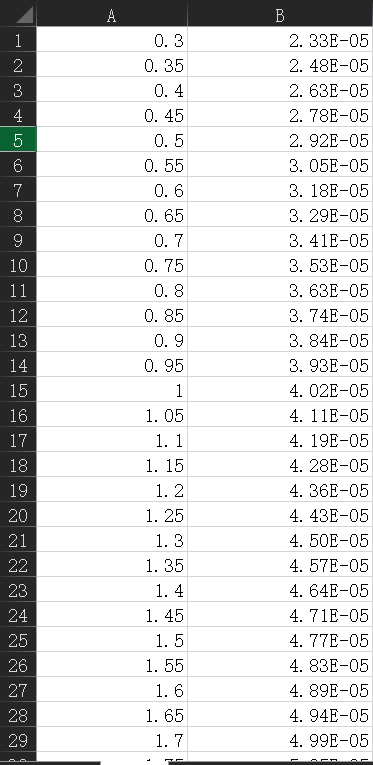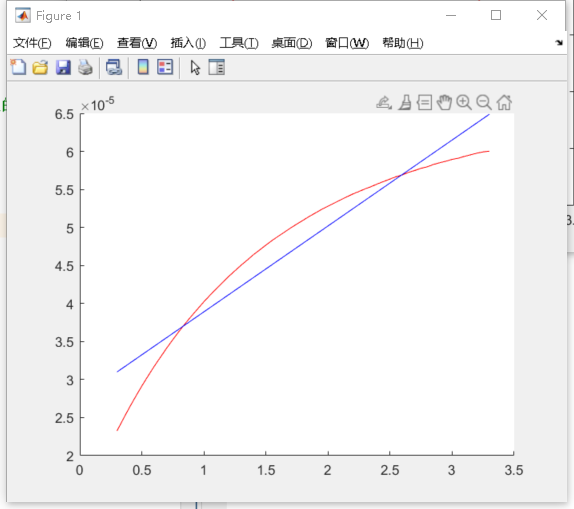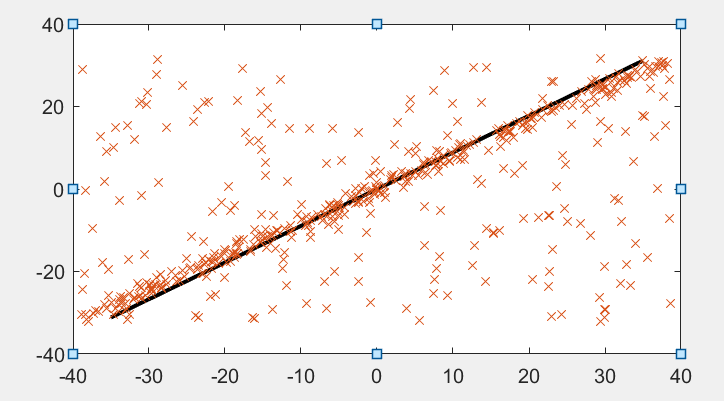• 给定若干一维点，进行线性差值，增加了正太噪声 Matlab代码 %% 初始数据准备 % 原始数据 x=[-60 -22 -6 0 11 15 70]; y=[0 0.43 1.6 1.89 1.65 0.64 0]; [~,num]=size(x);% 获取数据量 % 对x和y进行分段并且对应 ...
给定若干一维点，进行线性差值，增加了正太噪声
Matlab代码
%% 初始数据准备
% 原始数据
x=[-60 -22 -6 0 11 15 70];
y=[0 0.43 1.6 1.89 1.65 0.64 0];

[~,num]=size(x);% 获取数据量

% 对x和y进行分段并且对应
xx=[];
yy=[];
NumPts=150;
for i=1:(num-1)
newrow=linspace(x(i),x(i+1),NumPts);
newcol=linspace(y(i),y(i+1),NumPts);
xx=[xx,newrow];
yy=[yy,newcol];
end

% 添加随机噪声
[~,ysize]=size(yy);
amp=0.18;
% ynoise=amp*(rand(1,ysize)-1);
ynoise=normrnd(0,amp/6,1,ysize);
yy=yy+ynoise;
yy=yy-yy(1);
figure(1)
plot(xx,yy);




展开全文• 利用拉格朗日，调用分段线性差值函数，三次样条差值函数使用Matlab作图程序，使用超松弛迭代法解矩阵方程，定积分的计算，复合辛普森公式，复合梯形公式，求解常微分方程
• Matlab数据线性化 本文适用于小白。因为我是小白，所以找了半天才总结的一种求线性度的方法，整理成了m文件，导入数据后可以一键出图求线性度啦~ 事情是这样，我有一个如下形式的数据（Excel表格），需要将它拟合为...
Matlab数据线性化
本文适用于小白。因为我是小白，所以找了半天才总结的一种求线性度的方法，整理成了m文件，导入数据后可以一键出图求线性度啦~
事情是这样，我有一个如下形式的数据（Excel表格），需要将它拟合为一条直线然后求线性度。基本的思路：先用最小二乘法，对数据进行拟合。再求两条线的最大差值。最后根据线性度公式求得线性度。
在Matlab的里，有用最小二乘法生成拟合曲线的指令。如下。
[p,s]=polyfit(x,y,1);  %%% 数据拟合成直线
yy=polyval(p,x);

其中x对应A组数据，y对应B组，1表示拟合成一阶函数。修改这个可以拟合成高阶。求线性度需要的是直线，所以用的1阶。
我定义的yy，就是拟合后新的y。
拟合后的结果如下图：然后求得两条线的最大差值，再根据线性度公式就可以求线性度啦！
求最大差值的代码：
yc=abs(y-yy);   %% 差值的绝对值
m=max(yc);  %%求最大值

再根据线性度计算公式：
δ=ΔYmax/ Y*100%
将输出结果：
linear =

0.1291

结果是百分数，所以应该是12.91%。
代码连接放在下面，希望能帮到大家。
https://download.csdn.net/download/qq_41977160/16091282


展开全文数据建模
• 《用MATLAB实现拉格朗日插值和分段线性插值》由会员分享，可在线阅读，更多相关《用MATLAB实现拉格朗日插值和分段线性插值(6页珍藏版)》请...2) 会使用MATLAB软件进行拉格朗日插值算法和分段线性差值算法；3、实验原...
《用MATLAB实现拉格朗日插值和分段线性插值》由会员分享，可在线阅读，更多相关《用MATLAB实现拉格朗日插值和分段线性插值(6页珍藏版)》请在人人文库网上搜索。1、用MATLAB实现拉格朗日插值和分段线性插值1、 实验内容：用MATLAB实现拉格朗日插值和分段线性插值。2、 实验目的：1) 学会使用MATLAB软件；2) 会使用MATLAB软件进行拉格朗日插值算法和分段线性差值算法；3、实验原理：利用拉格朗日插值方法进行多项式插值，并将图形显式出来。4、实验步骤及运行结果(1)实现lagrange插值1)定义函数： f = 1/(x2+1) 将其保存在f.m 文件中，具体程序如下：function y = f1(x)y = 1./(x.2+1);2) 定义拉格朗日插值函数：将其保存在lagrange.m 文件中，具体实现程序编程如下：function y。2、 = lagrange(x0,y0,x)m = length(x); /区间长度/n = length(x0);for i = 1:nl(i) = 1;endfor i = 1:mfor j = 1:nfor k = 1:nif j = kcontinue;endl(j) = ( x(i) -x0(k)/( x0(j) - x0(k) )*l(j);endendendy = 0;for i = 1:ny = y0(i) * l(i) + y;end3) 建立测试程序，保存在text.m文件中，实现画图：x=-5:0.001:5; y=(1+x.2).-1; p=polyfit(x,y,n); 。3、py=vpa(poly2sym(p),10) plot_x=-5:0.001:5; f1=polyval(p,plot_x); figureplot(x,y,r,plot_x,f1)输入n=6,出现下面的图形：通过上图可以看到当n=6是没有很好的模拟。于是重新运行text.M并选择n=11由此可见n=11时的图像是可以很好的实现模拟(2)分段线性插值：建立div_linear.m文件。具体编程如下/*分段线性插值函数：div_linear.m 文件*/function y = div_linear(x0,y0,x,n)%for j = 1:length(x)for i = 1:n-1if (x。4、 = x0(i) & (x = x0(i+1)y = (x - x0(i+1)/(x0(i) - x0(i+1)*y0(i) + ( x - x0(i)/(x0(i+1) - x0(i)*y0(i+1);elsecontinue;endend%end测试程序(text2.m):n = input(输入n =:);x0 = linspace( -5,5,n);for x = -5:0.01:5y = div_linear(x0,f(x0),x,n);hold on;plot(x,y,r);plot(x,f(x),b);end2)运行测试程序，这是会出现：输入n=:2)输入n=6，并按Enter键。5、，出现：4)关掉图形界面后，重新运行程序，输入n=11，并按enter键后出现：5)再次关掉图形界面，输入n=100，并按enter键，出现：此时。图形将于原函数图形基本吻合，说明分割区间越多,图像接近真实的图像。(3)用lagrange插值观察y = |sin(k*x)|的误差分析：1)编写 函数文件，保存在f2.m 中x=0:0.01:1;k= input(输入k:)n= input(输入n:);y=abs(sin(k*pi*x);p=polyfit(x,y,n-1);py=vpa(poly2sym(p),8);plot_x=0:0.01:1;f1=polyval(p,plot_x);pl。6、ot(x,y,plot_x,f1); 2)运行该程序：输入k=:1输入n=:2出现如下图形界面：关掉图形界面后重新运行f2.m，输入k=：1，n=：3出现如下界面：再次关掉图形界面，输入k=：1，n=：6 后出现：此时图形基本吻合。类推，输入k=2， n=3后出现：k =2, n =11,出现如下图形：k =2,n =15,这时出现：k =2,n =19,出现：当k=2,n=21时，图形如下：此时基本吻合。5、实验总结：通过本次课程设计，我初步掌握了MATLAB运用，加深了对于各种线性插值的理解；培养了独立工作能力和创造力；综合运用专业及基础知识，解决实际数学问题的能力；在本次课程设计中，在老师的精心指导下，收益匪浅。同时对数学的研究有了更深入的认识。
展开全文• MATLAB 实现拉格朗日插值和分段线性插值 1 实验内容 用 MATLAB 实现拉格朗日插值和分段线性插值 2 实验目的 1 学会使用 MATLAB 软件 2 会使用 MATLAB 软件进行拉格朗日插值算法和分段线性 差值算法 3实验原理 ...
• 实验四 用 MATLAB 实现拉格朗日插值分段线性插值 一实验目的 1学会使用MATLAB 软件 2会使用MATLAB 软件进行拉格朗日插值算法和分段线性差值算法 二实验内容 1 用 MATLAB 实现 y = 1./(x^2+1; -1的拉格朗日插值分段...
• 实验四 用 MATLAB实现拉格朗日插值分段线性插值 一实验目的 1学会使用 MATLAB软件 2 会使用 MATLAB软件进行拉格朗日插值算法和分段线性差值算法 二实验内容 1 用 MATLAB实现 y = 1./(x^2+1; -1的拉格朗日插值分段...
• 实验四 用 MATLAB 实现拉格朗日插值分段线性插值 一实验目的 学会使用 MATLAB 软件 会使用 MATLAB 软件进行拉格朗日插值算法和分段线性差值算法 二实验内容 1 用 MATLAB 实现 y = 1./(x^2+1;-1的拉格朗日插值分段...
• 它是考虑了信息样品的形状、大小及与待估计块段相互间的空间位置等几何特征以及品位的空间结构之后，为达到线性、无偏和最小估计方差的估计，而对每一个样品赋与一定的系数，最后　进行加权平均来估计块段品位的方法...
• 实验四用MATLAE实现拉格朗日插值分段线性插值 一实验目的 )学会使用MATLAB软件 )会使用MATLAB软件进行拉格朗日插值算法和分段线性差值算法 二实验内容 1用MATLAB实现y二l./(x.A2+l; (-1二xUl )的拉格朗日插值分段...
• 选用matlab对实验数据进行处理 在实验过程中通过差值的计算可以看出x~v关系为线性关系所以选择使用MATLAB进行线性拟合 %输入实验数据 x=[4.2,4.3,4.4,4.4,4.4,4.7,4.8,4.9,5.0,5.1]%自变量位移 y=[-44,1,39,79,125,...
• MATLAB 实现拉格朗日插值和分段线性插值 1 实验内容 用MATLAB 实现拉格朗日插值和分段线性插值 2 实验目的 1 学会使用MATLAB 软件 2 会使用MATLAB 软件进行拉格朗日插值算法和分段线 性差值算法 3实验原理 利用...
• 1.我们初学matlab的时候，肯定会接触差值和拟合这两个知识点，因为这两个知识点对数据的而处理非常有用，今天呢我们来一起探讨一下如何从所得到的数据来实现简单的一维线性拟合。 2.下列步骤是我们此次算法的主要...
一维的线性拟合算法（matlab简单的ransac算法）
1.我们初学matlab的时候，肯定会接触差值和拟合这两个知识点，因为这两个知识点对数据的而处理非常有用，今天呢我们来一起探讨一下如何从所得到的数据来实现简单的一维线性拟合。
2.下列步骤是我们此次算法的主要思路：

Step 1 随机选取数据中的两个点，确定直线方程；
Step 2 计算数据点到直线的距离，统计距离小于一定阈值的点的数目记为count；
Step 3 重复Step1到Step 2一定次数，选取最大count值对应的直线为最终拟合的直线；

3.下面是所编写的matlab程序：
load('data_2.mat');%导入数据
x=Data(1,:);
y=Data(2,:);
count=zeros(1,100);
count(1)=0;
for j=2:100
a1=randperm(500,1);
b1=randperm(500,1);
x1=[x(a1) x(b1)];%产生一个随机的x点
y1=[y(a1) y(b1)];%产生一个随机的y点,与上面的x点产生呼应
a=polyfit(x1,y1,1);%得到上面两个点产生的一次方程的斜率和截距
k=a(1);b=a(2); %将斜率和截距的值赋给k和b
for i=1:500
p=abs(y(i)-k*x(i)-b)/sqrt(k*k+1);%计算每一个点到该直线的距离
if p<1
count(j)=count(j)+1;%得到距离小于1的点的个数
end
end
if count(j)>count(j-1);
x0=x1;y0=y1;
q=polyfit(x0,y0,1);
end
x5=(-35:0.1:35);
z=polyval(q,x5);
end
plot(x5,z,x,y,'x')

4.运行结果如下：我们可以看出这个拟合的效果还是不错的，希望这个简单的算法能够帮的到你哦~~


展开全文ransac算法
• 图像增强目的使得模糊图片变得更加清晰、图片模糊的原因是因为像素灰度差值变化不大，图片各区域产生视觉效果似乎都是一样的， 没有较为突出的地方，看起来不清晰的感觉解决这个问题的最直接简单办法，放大像素灰度...
• 图像增强目的使得模糊图片变得更加清晰、图片模糊的原因是因为像素灰度差值变化不大，如片各区域产生视觉效果似乎都是一样的， 没有较为突出的地方，看起来不清晰的感觉解决这个问题的最直接简单办法，放大像素灰度...
• matlab教程，PPT格式，包含 matlab基本知识、matlab入门、matlab作图、线性规划、无约束优化、非线性规划、统计工具箱、差值、微分方程等多项知识点，并且每个知识点独立成为PPT，内还含有matlab信号处理详解等文档...
• 二、单变量线性回归 2.1模型表示 同样以之前的房屋价格预测实例开始： 通过学习算法利用训练集训练模型h，对于新输入的数据size of house就可以输出其预测值price； 如何表达这个模型h： 2.2 cost损失...
• 差值主要算法 最近邻算法（一维插值） 拉格朗日插值算法（一维插值）...MATLAB实现插值 插值是在数据内部插值 interp1() interp1(x, y, xi, 'method') //x,y 插值节点 //xi 被插值点 /* method 使用的差值方法 默认...算法
• 本篇是线性规划系列中的最后一篇，讨论内点法（interior point method），相关算法在这里 原理本人也没有搞懂，所以本文的重点在于应用 内点法不能处理等式约束，只能处理不等式约束 由对偶的相关定理我们知道...
• 正版资源！我们用MATLAB的最主要的用途是来画图的，就像楼上说的MATLAB画图功能还是很强大的，一般的图应该都能解决。...MATLAB提供了比较强大的线性运算的工具，线性回归、差值、拟合等实现起来都很方便。
• 误差 导论 数值数组 程序设计 绘图 文件操作 非线性方程 常微分方程 差值 数值积分 线性方程组
• matlab的一些经典算法，比如解方程，绘图，线性规划，差值与拟合等等，一共10个小算法
• 利用Matlab，实现了影像增强、影像纠正，其中涉及到多界面以及之间参数调用，包含直方图均衡函数、直方图显示函数、间接法纠正算法以及双线性差值函数。
• 实验报告 实验项目名称 插值实验 所属课程名称 数学实验 实 验 类 型 综合性实验 实 验 日期 班 级 学 号 姓 名 成 绩 一实验概述 实验目的 掌握用Matlab插值的方法了解拉格朗日插值线性插值样条插值的基本思想了解...
• 班级_1250242_ 学号_125024202_ 姓名_侯继伟_ 1基点网平差实验 下图为三环路基点网各边段差值如图所示顺时针方向为正 假设各边段时间差均为1试采用线性方程组法求解平差系数要求用MATLAB编程求解并输出各环路的平差...
• 基于不在同一条直线上三点的抛物线插值函数。与Matlab中spline()在三个数据点时的结果一致。注意，大于三点的样条插值函数需要求解线性方程组，运算复杂，实时性较低。可参考Lapack及数值计算书籍
• 对图像进行缩放及旋转，采用不同的差值方法，并加以比较 matlab GUI做的数字图像处理。 1） 对图像放大2倍、5倍，采用最近内插、二次线性内插、三次内插，比较其效果。 2） 对图像依次旋转30°、60°，及一次性旋转...
• 具体如下：数据data_one = [time1, data1]data_two = [time2, data2]其中time1: 10~90time2：1~100线性差值：data = interp1(time1, data1, time2);因此，data的1~9，以及91~100，都是NAN判断哪些是NAN：用N...# matlab线性差值matlab 订阅